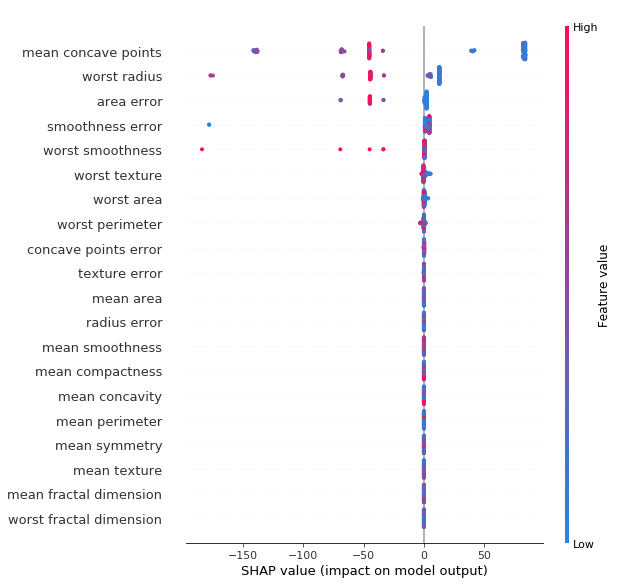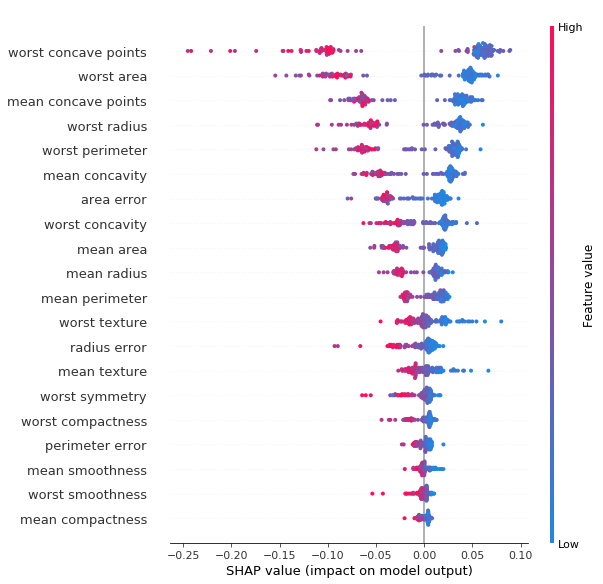In :
# Kod 1

import pandas as pd

from sklearn.tree import DecisionTreeClassifier
from sklearn.ensemble import RandomForestClassifier

from sklearn.model_selection import train_test_split

X = pd.DataFrame(breast_cancer["data"],
columns = breast_cancer["feature_names"])
y = pd.Series(breast_cancer["target"])

hyperparameters = {"criterion": 'gini',
"max_depth": 5,
"min_samples_leaf": 1,
"min_samples_split": 2,
"random_state": 42}

estimator_tree = DecisionTreeClassifier(random_state = hyperparameters["random_state"],
criterion = hyperparameters["criterion"],
max_depth = hyperparameters["max_depth"],
min_samples_leaf = hyperparameters["min_samples_leaf"],
min_samples_split = hyperparameters["min_samples_split"])

estimator_forest = RandomForestClassifier(random_state = hyperparameters["random_state"],
criterion = hyperparameters["criterion"],
max_depth = hyperparameters["max_depth"],
min_samples_leaf = hyperparameters["min_samples_leaf"],
min_samples_split = hyperparameters["min_samples_split"],
n_estimators = 100)

X_train, X_test, y_train, y_test = train_test_split(X, y,
test_size = 0.25,
random_state = 42)
estimator_tree.fit(X = X_train, y = y_train)
estimator_forest.fit(X = X_train, y = y_train)

Out:
RandomForestClassifier(bootstrap=True, class_weight=None, criterion='gini',
max_depth=5, max_features='auto', max_leaf_nodes=None,
min_impurity_decrease=0.0, min_impurity_split=None,
min_samples_leaf=1, min_samples_split=2,
min_weight_fraction_leaf=0.0, n_estimators=100, n_jobs=1,
oob_score=False, random_state=42, verbose=0, warm_start=False)
In :
# Kod 2

import shap
explainer_tree = shap.TreeExplainer(estimator_tree)
shap_values_tree = explainer_tree.shap_values(X_test)
shap.summary_plot(shap_values_tree, X_test)In :
explainer_forest = shap.TreeExplainer(estimator_forest)
shap_values_forest = explainer_forest.shap_values(X_test)
shap.summary_plot(shap_values_forest, X_test)In :
from sklearn.metrics import accuracy_score

In :
print(accuracy_score(y_true = y_test, y_pred = estimator_tree.predict(X_test)))
print(accuracy_score(y_true = y_test, y_pred = estimator_forest.predict(X_test)))

0.958041958041958
0.965034965034965

In :
print(y_train.value_counts())
print(y_test.value_counts())

1    268
0    158
dtype: int64
1    89
0    54
dtype: int64

In [ ]: